# Error in this solution?

Homework Helper

## Homework Statement

Find ##v_o(t)## and plot it.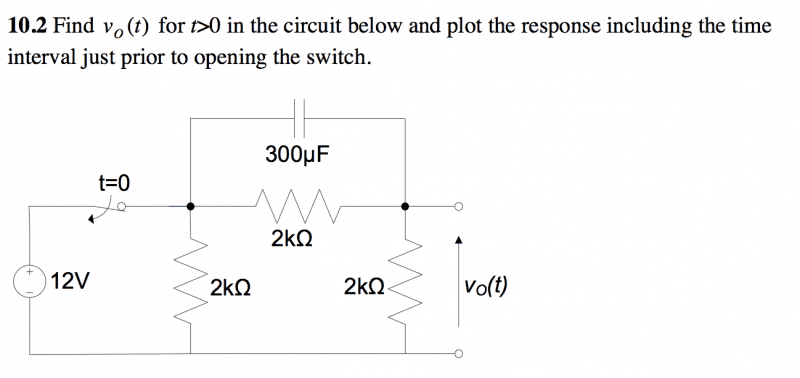## The Attempt at a Solution

The solution was provided, and I got all of the same numbers except for one thing. For some reason, in step 3 there is a negative sign in front of ##v_c(t = 0^+)## when calculating ##v_o(t = 0^+)## using a voltage divider.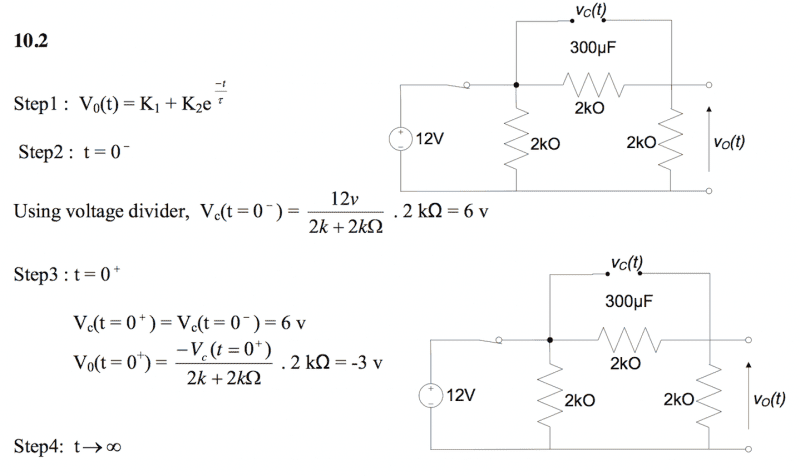Could someone shed some light to where that came from or is it simply an error?

gneill
Mentor
Mark the polarity of the capacitor charge for time t < 0. When the switch opens at t = 0, what direction will the current flow through the "output" resistor? So what's the resulting direction of potential drop?

Homework Helper
Mark the polarity of the capacitor charge for time t < 0. When the switch opens at t = 0, what direction will the current flow through the "output" resistor? So what's the resulting direction of potential drop?

So you mean the capacitor discharges like so: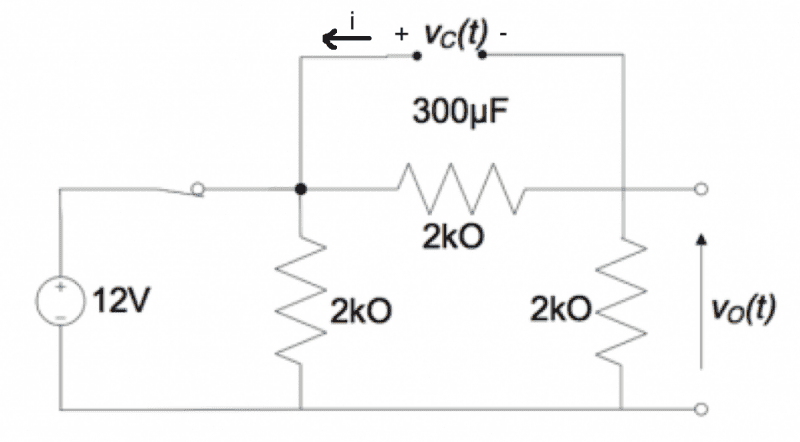So the current will flow head to tail through the 2k.

gneill
Mentor
Draw the current through each component.

Homework Helper
Draw the current through each component.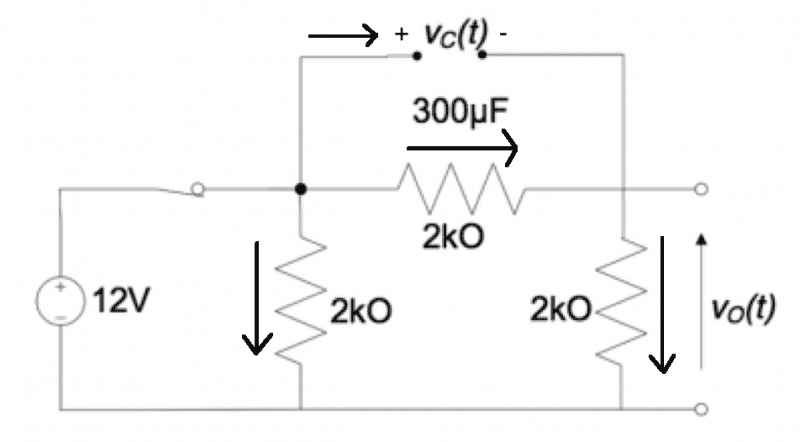I hope that looks okay now.

gneill
Mentor
You have both currents through the 2k resistors heading downwards into the same node. Where does the current go? (think KCL)

Homework Helper
You have both currents through the 2k resistors heading downwards into the same node. Where does the current go? (think KCL)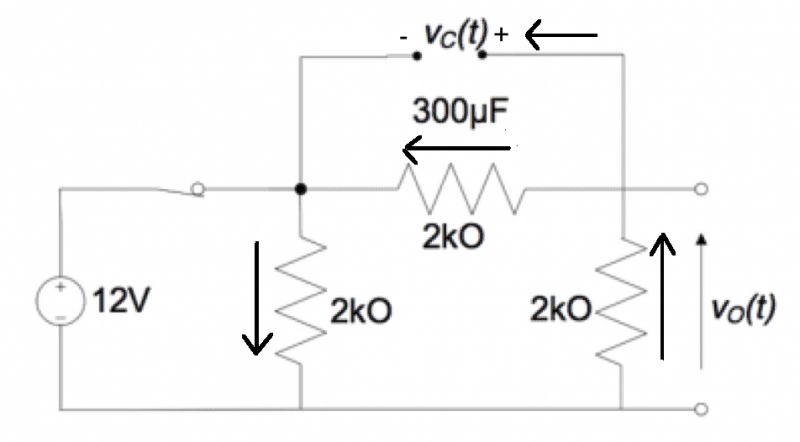I'm honestly a little confused if that's not the case.

gneill
Mentor
You're making things worse! You've changed the polarity of the charge on the capacitor for no reason. Your original figure was correct: the capacitor was charged (at steady state) to the potential difference across the 2k resistor in parallel with it.

Current flows from higher potential (+) to lower potential (-). Current leaving the + terminal of the capacitor flows around the circuit to make its way back to the - terminal of the capacitor. You will not find current heading away from the - terminal of the capacitor!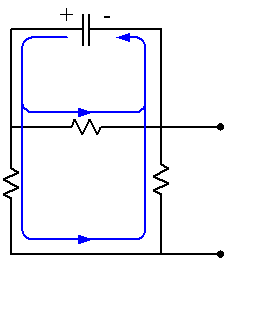•STEMucator
Homework Helper
You're making things worse! You've changed the polarity of the charge on the capacitor for no reason. Your original figure was correct: the capacitor was charged (at steady state) to the potential difference across the 2k resistor in parallel with it.

Current flows from higher potential (+) to lower potential (-). Current leaving the + terminal of the capacitor flows around the circuit to make its way back to the - terminal of the capacitor. You will not find current heading away from the - terminal of the capacitor!

View attachment 76332

I realize now that the voltage source was completely messing with my head, even though at ##t = 0^+## I shouldn't even be considering it! Only the current from the capacitor should matter at that point and the way it discharges is given by it's polarity (which can be found by looking at the battery terminals at ##t = 0^-##).

Thank you for clarifying this for me. Fooled once, never fooled again.